# A Ray Tracer - Part 2

### Tags: C, Graphics, Mathematics, Programming, Ray Tracing

Last time we build up the basics of the raytracer: some essential math and the corresponding function implementations and structures in the C programming language. We will expand the ray tracer this time to generate actual graphics and to generate these images with multiple spheres, light sources, and reflections.If you compiled and ran the example attached to the last article, you should have noticed a console output which looks like this: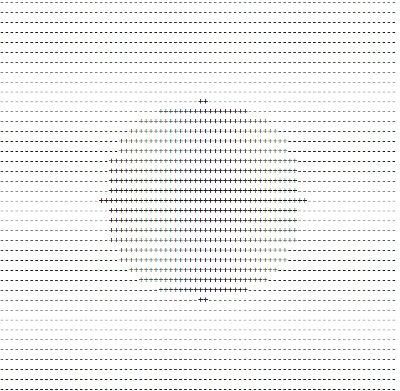Not super exciting, I know, but it verifies the correctness of our ray/sphere intersect function. Let's take this one step further and create an actuall graphic which you can open in an image viewer.

Computer images are built up out of pixels (picture elements). Each pixel can represent any colour made up from the three primary colours red, green and blue. When we say that an image is '24-bit colour', we mean that each pixel is built up out of 3 values, each 8 bit long. In this way, we can let the red, green and blue component vary in value between 0 and 255 because. With 24 bit colour, we have enough to represent over 16.7 million colours.

Let's start with something simple. Suppose we want to programatically create the Finnish flag: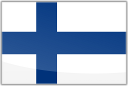The first thing we need to do is agree on the format we're going to use. There are many image formats such as jpeg, png, gif, etc. Most of these are quite complex to generate unless you use existing libraries. For this tutorial however, we will use something very simple: the ppm (portable pixmap) format. The ppm format is a simple uncompressed format from the Netpbm family of image formats. The PPM format has the following components (also called the header) needed for the file to be identified and displayed correctly by the image viewer:

• A "magic number" identifying the file type, in our case this will be "P6"
• Whitespace (any TAB/SPACE/NEWLINE)
• Width of the image, ASCII decimal representation
• Whitespace (any TAB/SPACE/NEWLINE)
• Height of the image, ASCII decimal representation
• Whitespace (any TAB/SPACE/NEWLINE)
• Maximum colour value, ASCII decimal representation
• Whitespace (single character, usually newline)
• Actual image data

Everything up until the image data can be written in C as follows:

fprintf(f, "P6 %d %d %d\n", width, height, 255);

Where 'f' is the pointer to an opened and writeable file. We will get back to this later. 'P6' in the PPM format indicates 'raw' PPM format. You can read more about the details in the links mentioned above.

So what is the image data composed of? It simply is the colour information per pixel, for all the pixels in the image. In other words it is the data represented by 3 bytes per pixel (8 bit per colour, 24 bit total) multiplied by the width and the height of the image. Therefor, a 24 bit image, measuring 800 pixels wide with a height of 600 pixels will contain 3 x 800 x 600 bytes of data, making 1.44MB of data. Interesting note: this is the amount of data you could store on a standard PC formatted double sided high density 3 1/2 inch floppy disk.

To open a PPM file, you need to install an appropriate viewer since Microsoft Windows does not come with PPM support by default. Linux should be able to open it out of the box. For Windows (and Mac) users, I've found these programs that should be fien for displaying PPM images:

For Windows: http://www.irfanview.com/

To write a PPM file, we can write a function in C which looks like this:

/* Output data as PPM file */
void saveppm(char *filename, unsigned char *img, int width, int height){

/* FILE pointer */
FILE *f;

/* Open file for writing */
f = fopen(filename, "wb");

/* PPM header info, including the size of the image */
fprintf(f, "P6 %d %d %d\n", width, height, 255);

/* Write the image data to the file - remember 3 byte per pixel */
fwrite(img, 3, width*height, f);

/* Make sure you close the file */
fclose(f);

}

The pointer *img points to our image data, which consists of 3*width*height bytes of data, representing each pixel with its three colour components. Since each colour component can vary between 0 and 255, we can easly create the two colours needed for our flag. White can be represented with each component fully on, that is r=255, g=255, and b = 255. Similarly, the colour blue could be r=0, g=0, and b=128. This will give one shade of blue; you could use others by varying the value of each component.

The image data itself we can store in an array. Later on we can use dynamic memory allocation, but for now, a statically allocated array will do fine. Inside this array, we have to set the correct pixels white and blue in order to reporesent the Finnish flag. This is a matter of pretending you're in front of a canvas and drawing certain sections in a certain colour.

In C, we could do this as follows:

#define WIDTH  800
#define HEIGHT 500

int main(int argc, char *argv[]){

unsigned char img[WIDTH * HEIGHT * 3];
int i,j;

for(i=0; i<HEIGHT; i++){
for(j=0; j<WIDTH; j++){
if( (j>=250 && j<350) || (i>=200 && i<300) ){
img[(j + i*WIDTH)*3 + 0] = 0;
img[(j + i*WIDTH)*3 + 1] = 0;
img[(j + i*WIDTH)*3 + 2] = 128;
}else{
img[(j + i*WIDTH)*3 + 0] = 255;
img[(j + i*WIDTH)*3 + 1] = 255;
img[(j + i*WIDTH)*3 + 2] = 255;
}
}

}

saveppm("image.ppm", img, WIDTH, HEIGHT);

return 0;
}

Within each iteration of the inner for loop, we draw one pixel. After one complete cycle of the outer for loop, one horizontal line is fully drawn. Within the inner for loop, we decide the colour each pixel will have, and set it's triplet (r,g,b) to the correct values. Whether a pixel becomes blue or wite is defined by the if statement in the inner for loop. It just checks if we are in the area of the flag that needs to be blue or white, calculated in advance knowing the width and height of the image. At the end, the image is written to a file, and you should be able to open it with an image viewer. The full version of this program is attached to this post (flag1.c).

Back to our ray tracer. We can now go back to the program we made last time and, instead of generating an ASCII output with a couple of printf() statements we can colour our sphere in any colour we want. We can also generate an image that is much larger than the 40x40 'image' we created before. As an example, let's build an 800x600 image with a red sphere on a black background. The resulting program is also attached (raytrace_sphere_1.c).

Hardly realistic, but it provides a start to make things look much better. The reason why it doesn't look realistic is because we're missing probably the most important element of a scene: "Lights, Camera, Action". We have the camera and the action (the sphere) - now all we need is light! First thing we want to do is change the way colour is assigned. After all, colour is a property of the object in question and should hence be assigned as such. We can change our code a little so that we have an element called 'colour' which contains the three colour components of the final shade. We can easily do this again with a structure:

/* Colour definition */
typedef struct{
float red, green, blue;
}colour;

Why floats? We're going to need a way to represent the colour as a precentage. While one could calculate in absolute values of 0 to 255, it is easier to be able to say "colour this object with 50% red, 30% green and 10% blue". Floating points allow us to do that easily by specifying a value between 0.0 and 1.0, which represent the percentages.

As we said before, we need lights. Lights within this context have two things: a position in 3D space and an intensity per colour component. This we can represent as follows:

/* Light definition */
typedef struct{
vector pos;
colour intensity;
}light;

We want to be able to create a couple different materials and assign these to the sphere. A material in this context will be defines as an entity that can be assigned to an object, defining its reflectivity (0% - 100%) and diffuse colour. Diffuse colour will indicate the colour the object will reflect when being lit. Reflectivity will be used to determine how 'shiny' an object is and will act as a mirror for other objects. A material can be constructed as:

/* Material definition */
typedef struct{
colour diffuse;
float reflection;
}material;

Similarly, our sphere object can now become:

/* Sphere Primitive definition */
typedef struct{
vector pos;
int material;
}sphere;

Here, material is the id which will be used to select the assigned material from an array of materials. The idea now, simplified, is to shoot our ray into the scene, and should it hit the sphere, bounce it off of the sphere (reflect) and check if it hits the lightsource. If it does, this point on the sphere is lit, otherwise it is not.

We now have to develop a way to use these newly defined entities. Let's start with building up a scene. We have several spheres we want to show (instead of just the one from the previous example). We also want to position some lightsources, since one lightsource will not be sufficient to provide realistic lighting. For now we will go through the process of positioning them one by one, but later on it would be easier to use a text file describing the scene to set this up. We will start with three materials, three lightsources and three spheres, all in their proper arrays. I'm not going to paste the code for that here since it's quite long and repetitive, but you can see the code in question, together with the next couple of topics in raytrace_sphere_1.c attached to this post.

The next thing we should do is to revise our ray-sphere intersection function. The way it is currently written it will only return true or false indicating intersection or not. We should however be able to find out exactly where this point of intersection is. Moreover, in case there are two intersection points, we want to know the one closest to the screen. That is the one that we actually see - the other one is on the other side of the sphere, and not visible. To accomplish this, we can modify the function as follows:

/* Check if the ray and sphere intersect */
bool intersectRaySphere(ray *r, sphere *s, float *t){

bool retval = false;

/* A = d.d, the vector dot product of the direction */
float A = vectorDot(&r->dir, &r->dir);

/* We need a vector representing the distance between the start of
* the ray and the position of the circle.
* This is the term (p0 - c)
*/
vector dist = vectorSub(&r->start, &s->pos);

/* 2d.(p0 - c) */
float B = 2 * vectorDot(&r->dir, &dist);

/* (p0 - c).(p0 - c) - r^2 */

/* Solving the discriminant */
float discr = B * B - 4 * A * C;

/* If the discriminant is negative, there are no real roots.
* Return false in that case as the ray misses the sphere.
* Return true in all other cases (can be one or two intersections)
*  t, the solution to the equation represents the closest point
* where our ray intersects with the sphere.
*/
if(discr < 0)
retval = false;
else{
float sqrtdiscr = sqrtf(discr);
float t0 = (-B + sqrtdiscr)/(2);
float t1 = (-B - sqrtdiscr)/(2);

/* We want the closest one */
if(t0 > t1)
t0 = t1;

/* Verify t0 larger than 0 and less than the original t */
if((t0 > 0.001f) && (t0 < *t)){
*t = t0;
retval = true;
}else
retval = false;
}

return retval;
}

The value 't' represents the length of the closest intersection. We can illustrate this as follows: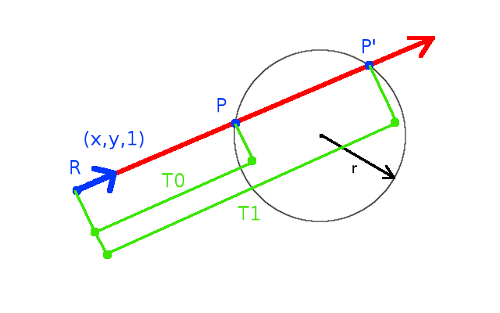The value 't' is a scalar, and in order to find the vector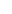, we have to write a function which takes this scalar and multiplies it with our vectorwhich is the direction of our ray. Multiplication of a vector with a scalar is just multiplying each component with the scalar. We know this already from part 1, in which we discussed parameterizing. Since the direction of our ray is always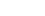, the scalar multiplication will result in a scaled vector representing the distance from the start of our ray to the point of impact on the sphere. Adding this scaled vector to the start position of our ray will give the vector. One thing I'd like to note here is the check whether t greater than 0, which is written as t0 > 0.001f in the code. The reason for using 0.001 instead of 0 has to do with floating point comparison and precision when representing floating point numbers. You can read more about this here.

We will need two additional functions in our arsenal to deal with the above: scaling and adding vectors:

/* Calculate Vector x Scalar and return resulting Vector*/
vector vectorScale(float c, vector *v){
vector result = {v->x * c, v->y * c, v->z * c };
return result;
}

/* Add two vectors and return the resulting vector */
vector result = {v1->x + v2->x, v1->y + v2->y, v1->z + v2->z };
return result;
}

Now that we have materials, spheres and lights, it's time to start working on the main objective. We need to simulate how light interacts with the spheres and the camera to build a realistic image. Each point on each sphere can be lit or unlit. The points that are lit can be lit with different intensities depending on how much light reaches the point in question. With our ray which we shoot through the screen will need to determine if it hits a point on one of the spheres, if light reaches this point. Furthermore, light reflects of off objects so from the impact point we reflect the ray and perhaps hit another sphere.

﻿Our algorithm will look, simplified, like this:

for(HEIGHT){
for(WIDTH){
do for every ray:
- Find closest ray/sphere intersection:
* Iterate over every sphere

- Check if we reach a lightsource from this point
* Iterate over every lightsource
* Find right colour

- Either go with reflected ray or go to next pixel
}
}

To get the colour and intensity of each pixel, we will apply Lambertian reflectance to model diffuse reflection whereby the light will be reflected in equally in all directions from the point where the light hits the object. This is a very simple model where: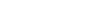. Here,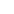is the normal at the point of intersection, anddenotes the normalized vector from the point of intersecton to the light source.represents the colour of the sphere and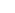is the intensity of the incoming light. The resulting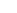scalar represents the surface brightness at that point.

We will program this later as follows:

﻿- For each lightsource that can be reached:
* Calculate Lambert dot product with material reflection
* Calculate each colour component, consisting of:
- Lambert dot product result
- Per colour intensity of the incoming light

We then need to get the reflection of our incoming ray and see if we hit another sphere and repeat the process. Getting the reflected ray is simple according to the law of reflection :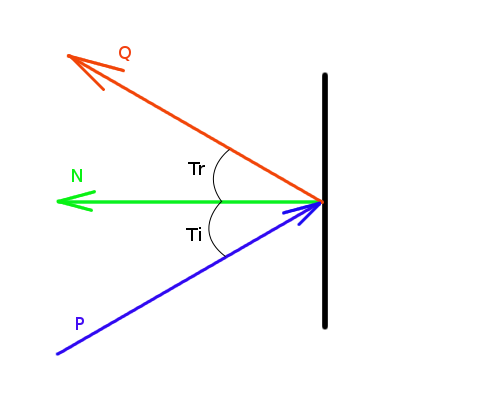The angle between the incoming ray and the normal is equal to the angle between the reflected ray and the normal (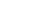). The normal on a point on a sphere is a vector which can be constructed by drawing it starting at the center of the sphere and going through our point of intersection. Mathematically, we can determine this when we present the situation as in this drawing:When we assume thatandare normalised, we know from basic trigonometry that:From the drawing above, we can also see that:After summation, we can get the direction: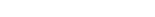We can work this further out. We know that: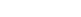.

Using orthogonal projection:We know thatfor some scalar a. Since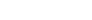is orthogonal to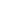, we have that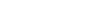.

Multiply out the dot product and solving for a gives: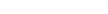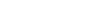.

The result is that:From the definition of the dot product, we know that.

Applying this to our situation gives that, withour Normal: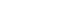Knowing thatThis gives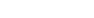Coming back to:, we can solve this as follows:This can be solved further as: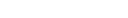That's it - we now have our reflected vector and continue to the next ray trace loop with this vector as a start.

The full C code can be found in the raytrace_sphere_2.c file attached. The main raytrace loop will continue to iterate over multiple reflections until a certain level (otherwise it might run forever). We can further enhance this program by adding shadows to the final image. Calculating shadows is pretty easy: one just has to trace the 'lightRay' found before determining the colour back to each object. If it intersects with another sphere, the point in question sits in the shadow of said sphere. The solution to this is in the attached raytrace_sphere_3.c.

### Ray tracing on Z-80

Thank you for a great tutorial. A lot of the math is at the upper edge of my memory/knowledge. I graduated High School in 1984 and only learned enough trig for Electronics AC Analysis since then.

But I have a Z-80 Single Board computer I like pushing to it's limits.

It has 32k ROM for firmware and 32k RAM for data. From a quick read of the ppm format, an image 100X75X3 bytes = 22,500 bytes plus the header.

Also, I'm not sure I want to include the full stdio, stdbool and math libs in my executable. bool should be easy enough to include. Not sure how much of math I'd need to include, but I should be able to work that out. The tricky part, as I see it, is saving the ppm file without stdio.

But I'm gonna keep reading over your great tutorial until I figure out the math in my head.

Cheers,

John Gay

### RE: Ray tracing on Z-80

Hi!

I'm glad you found the tutorial useful! You can probably get away with simplifying this a lot to get it to work on a Z-80.

Johan.

### So far neither c compiler for

So far neither c compiler for the Z-80 allows passing struct's to functions or using them as return types.

And I'm not really a programmer, I just like seeing how code works.

Another idea I've been tossing around for many decades was to make a ray tracer that can handle 4 or more dimensions. But I want to take the 3D slice of the higher dimensional objects and trace the resulting 3D scene. I think I might understand enough of your code to add a 4th dimension. But I'll try your more complete ray tracer since i'll need triangles for the cubes and other 4D objects.

Still loving how simple and easy to understand your code and tutorials are.

Cheers,

John Gay

### RE: So far neither c compiler for

They're just pointers - that should not be an issue. Could you let me know exactly what the problem is? This is interesting!

It's been decades since I did anything with a Z-80, so I'm afraid I can't help you with specifics.

Johan.

### They are not supported in

They are not supported in SDCC or z88dk.

QUOTE

~3.1 Standard-Compliance
SDCC aims to be a conforming freestanding implementation of the C programming language.
3.1.1 ISO C90 and ANSI C89
Use --std-c89 to compile in this mode.
The latest publicly available version of the standard ISO/IEC 9899 - Programming languages - C should be
available at: http://www.open-std.org/jtc1/sc22/wg14/www/standards.html#9899.
Deviations from standard compliance:
 structures and unions cannot be passed as function parameters and cannot be a return value from a function, e.g.:
struct s { ... };
struct s foo1 (struct s parms) /* invalid in SDCC although allowed in ANSI */
{
struct s rets;
...
return rets; /* is invalid in SDCC although allowed in ANSI
*/
}

/QUOTE

Although z88dk did try to convert the struct's to pointers, that failed.

Maybe if they werre converted to actual pointers it might work.

Cheers,

John Gay

### RE: They are not supported in

Interesting. Yes, you should just be able to make them actual pointers.

Johan.

### So I found this example for

So I found this example for pointers to structures:

https://overiq.com/c-programming-101/structures-and-functions-in-c/

but although I can get the examples in this tutorial to compile with z88dk, I cannot get my head around converting your functions to using pointers to struct's?

/* Subtract two vectors and return the resulting vector */

vector vectorSub(vector *v1, vector *v2){

vector result = {v1->x - v2->x, v1->y - v2->y, v1->z - v2->z };

return result;

}

which compiles for in gcc, but with z88dk I get:

$zcc +z80 -vn -clib=new raytrace_sphere_Z.c -o raytrace_sphere_Z -lm -create-app raytrace_sphere_Z.c:53:17: warning: Can't define a function returning an struct, converting to a pointer [-Wconversion] raytrace_sphere_Z.c:54:21: fatal error: Unknown symbol: v1 Compilation aborted which is here: /* We need a vector representing the distance between the start of * the ray and the position of the circle. * This is the term (p0 - c) */ vector dist = vectorSub(&r->start, &s->pos); So I don't know how to convert this to passing pointers instead of structs? I've tried adding '*' but it still fails. I.E.: /* Subtract two vectors and return the resulting vector */ vector *vectorSub(vector *v1, vector *v2){ vector *result = {v1->x - v2->x, v1->y - v2->y, v1->z - v2->z }; return result; } ... /* We need a vector representing the distance between the start of * the ray and the position of the circle. * This is the term (p0 - c) */ vector *dist = vectorSub(&r->start, &s->pos); but it still fails and I don't understand where I am going wrong? Sorry to keep asking, but I would really like to see this work. Cheers, John Gay ### Re: So I found this example for Hi, Your second example won't work because you don't actually create the storage, just a pointer. It's also not a good idea to return addresses of local variables. You could do the following instead: Change the function as follows: void vectorSub(vector *v1, vector *v2, vector *result){ result->x = v1->x - v2->x; result->y = v1->y - v2->y; result->z = v1->z - v2->z; } Call it e.g: vector a = {4,5,6}; vector b = {1,2,3}; vector c; vectorSub(&a,&b,&c); Now c will have the resulting subtraction of a and b. Johan ### Thank you for the help. I Thank you for the help. I have converted all the functions in raytrace_sphere_2.c that returned sctructures according to your example and got it to compile cleanly with gcc again. Now when I compile with zcc, I get:$ zcc +z80 -vn -clib=new raytrace_sphere_Z.c -o raytrace_sphere_Z -lm -create-app
raytrace_sphere_Z.c:260:36: fatal error: Unknown symbol: materials
Compilation aborted

which is the line:

/* Find the material to determine the colour */

material currentMat = materials[spheres[currentSphere].material];

so Im not sure why? This is the array of materials for the objects to be rendered.

Unfortunately, zcc stops at the first error so I can only work one problem at a time.

Thank you so much for your continued support!

Cheers,

John Gay

### Re: Thank you for the help. I

Try to hard-code a material at that location. Something like:

material currentMat; //= materials[spheres[currentSphere].material];
currentMat.diffuse.red = 1;
currentMat.diffuse.green = 0;
currentMat.diffuse.blue = 0;
currentMat.reflection = 0.2;

### That worked, and now I'm

That worked, and now I'm getting the same error for lights.

I guess that means that zcc doesn't support arrays? That is going to make this a lot harder.

But thanks again for your help. At least I (you) are making progress (-=

Cheers,

John Gay

### Re: That worked, and now I'm

Maybe it has problems with arrays of structs? Also, could it be that the array is out of scope (too far from where it is declared and initialised)?

In any case, there are ways around it that shouldn't be too painful. You could even just define them in a header file or something instead of making them structs/arrays.

Johan,

### Thanks a lot !!

There are many ray tracing tutorials on the internet but this is the most straightforward and easy to understand I've found. Thank you !!

### Re: Thanks a lot !!

Thank you! Glad you found it useful!

### doubt

Hi, thank you so much for making ray tracing easy to understand!

I was wondering... how can I add more spheres to the code raytrace_sphere_2.c

Thank you !!!!

Hi,

To add a sphere, have a look at the place in main() where you will find 'sphere spheres;'  You can add one there (3 becomes 4), and after that set some coordinates for it and assign material:

	spheres.pos.x = 100;
spheres.pos.y = 100;
spheres.pos.z = 0;
spheres.material = 1;

### Hi, thank you very for

Hi, thank you very for answering!

These are the changes I made to the code but its not outputting any file, I am very sorry for the inconvenience but I just started to learn about coding and raytracing :)

Thank You

/* A simple ray tracer */

#include <stdio.h>
#include <stdbool.h> /* Needed for boolean datatype */
#include <math.h>

#define min(a,b) (((a) < (b)) ? (a) : (b))

/* Width and height of out image */
#define WIDTH  800
#define HEIGHT 600

/* The vector structure */
typedef struct{
float x,y,z;
}vector;

/* The sphere */
typedef struct{
vector pos;
int material;
}sphere;

/* The ray */
typedef struct{
vector start;
vector dir;
}ray;

/* Colour */
typedef struct{
float red, green, blue, orange;
}colour;

/* Material Definition */
typedef struct{
colour diffuse;
float reflection;
}material;

/* Lightsource definition */
typedef struct{
vector pos;
colour intensity;
}light;

/* Subtract two vectors and return the resulting vector */
vector vectorSub(vector *v1, vector *v2){
vector result = {v1->x - v2->x, v1->y - v2->y, v1->z - v2->z };
return result;
}

/* Multiply two vectors and return the resulting scalar (dot product) */
float vectorDot(vector *v1, vector *v2){
return v1->x * v2->x + v1->y * v2->y + v1->z * v2->z;
}

/* Calculate Vector x Scalar and return resulting Vector*/
vector vectorScale(float c, vector *v){
vector result = {v->x * c, v->y * c, v->z * c };
return result;
}

/* Add two vectors and return the resulting vector */
vector result = {v1->x + v2->x, v1->y + v2->y, v1->z + v2->z };
return result;
}

/* Check if the ray and sphere intersect */
bool intersectRaySphere(ray *r, sphere *s, float *t){

bool retval = false;

/* A = d.d, the vector dot product of the direction */
float A = vectorDot(&r->dir, &r->dir);

/* We need a vector representing the distance between the start of
* the ray and the position of the circle.
* This is the term (p0 - c)
*/
vector dist = vectorSub(&r->start, &s->pos);

/* 2d.(p0 - c) */
float B = 2 * vectorDot(&r->dir, &dist);

/* (p0 - c).(p0 - c) - r^2 */

/* Solving the discriminant */
float discr = B * B - 4 * A * C;

/* If the discriminant is negative, there are no real roots.
* Return false in that case as the ray misses the sphere.
* Return true in all other cases (can be one or two intersections)
* t represents the distance between the start of the ray and
* the point on the sphere where it intersects.
*/
if(discr < 0)
retval = false;
else{
float sqrtdiscr = sqrtf(discr);
float t0 = (-B + sqrtdiscr)/(2);
float t1 = (-B - sqrtdiscr)/(2);

/* We want the closest one */
if(t0 > t1)
t0 = t1;

/* Verify t1 larger than 0 and less than the original t */
if((t0 > 0.001f) && (t0 < *t)){
*t = t0;
retval = true;
}else
retval = false;
}

return retval;
}

/* Output data as PPM file */
void saveppm(char *filename, unsigned char *img, int width, int height){
/* FILE pointer */
FILE *f;

/* Open file for writing */
f = fopen(filename, "wb");

/* PPM header info, including the size of the image */
fprintf(f, "P6 %d %d %d\n", width, height, 255);

/* Write the image data to the file - remember 3 byte per pixel */
fwrite(img, 3, width*height, f);

/* Make sure you close the file */
fclose(f);
}

int main(int argc, char *argv[]){

ray r;

material materials;
materials.diffuse.red = 1;
materials.diffuse.green = 0;
materials.diffuse.blue = 0;
materials.diffuse.orange = 0;
materials.reflection = 0.2;

materials.diffuse.red = 0;
materials.diffuse.green = 1;
materials.diffuse.blue = 0;
materials.diffuse.orange = 0;
materials.reflection = 0.5;

materials.diffuse.red = 0;
materials.diffuse.green = 0;
materials.diffuse.blue = 1;
materials.diffuse.orange = 0;
materials.reflection = 0.7;

materials.diffuse.red = 0;
materials.diffuse.green = 0;
materials.diffuse.blue = 0;
materials.diffuse.orange = 1;
materials.reflection = 0.9;

sphere spheres;
spheres.pos.x = 50;
spheres.pos.y = 30;
spheres.pos.z = 0;
spheres.material = 0;

spheres.pos.x = 200;
spheres.pos.y = 400;
spheres.pos.z = 0;
spheres.material = 1;

spheres.pos.x = 500;
spheres.pos.y = 140;
spheres.pos.z = 0;
spheres.material = 2;

spheres.pos.x = 100;
spheres.pos.y = 100;
spheres.pos.z = 0;
spheres.material = 1;

light lights;

lights.pos.x = 0;
lights.pos.y = 240;
lights.pos.z = -100;
lights.intensity.red = 1;
lights.intensity.green = 1;
lights.intensity.blue = 1;
lights.intensity.orange = 1;

lights.pos.x = 3200;
lights.pos.y = 3000;
lights.pos.z = -1000;
lights.intensity.red = 0.6;
lights.intensity.green = 0.7;
lights.intensity.blue = 1;
lights.intensity.orange = 0.8;

lights.pos.x = 600;
lights.pos.y = 0;
lights.pos.z = -100;
lights.intensity.red = 0.3;
lights.intensity.green = 0.5;
lights.intensity.blue = 1;
lights.intensity.orange = 0.4;

lights.pos.x = 500;
lights.pos.y = 0;
lights.pos.z = -200;
lights.intensity.red = 0.3;
lights.intensity.green = 0.5;
lights.intensity.blue = 1;
lights.intensity.orange = 1;

/* Will contain the raw image */
unsigned char img[3*WIDTH*HEIGHT];

int x, y;
for(y=0;y<HEIGHT;y++){
for(x=0;x<WIDTH;x++){

float red = 0;
float green = 0;
float blue = 0;
float orange = 0;

int level = 0;
float coef = 1.0;

r.start.x = x;
r.start.y = y;
r.start.z = -2000;

r.dir.x = 0;
r.dir.y = 0;
r.dir.z = 1;

do{
/* Find closest intersection */
float t = 20000.0f;
int currentSphere = -1;

unsigned int i;
for(i = 0; i < 4; i++){
if(intersectRaySphere(&r, &spheres[i], &t))
currentSphere = i;
}
if(currentSphere == -1) break;

vector scaled = vectorScale(t, &r.dir);

/* Find the normal for this new vector at the point of intersection */
vector n = vectorSub(&newStart, &spheres[currentSphere].pos);
float temp = vectorDot(&n, &n);
if(temp == 0) break;

temp = 1.0f / sqrtf(temp);
n = vectorScale(temp, &n);

/* Find the material to determine the colour */
material currentMat = materials[spheres[currentSphere].material];

/* Find the value of the light at this point */
unsigned int j;
for(j=0; j < 4; j++){
light currentLight = lights[j];
vector dist = vectorSub(&currentLight.pos, &newStart);
if(vectorDot(&n, &dist) <= 0.0f) continue;
float t = sqrtf(vectorDot(&dist,&dist));
if(t <= 0.0f) continue;

ray lightRay;
lightRay.start = newStart;
lightRay.dir = vectorScale((1/t), &dist);

unsigned int k;
for (k = 0; k < 4; ++k) {
if (intersectRaySphere(&lightRay, &spheres[k], &t)){
break;
}
}
/* Lambert diffusion */
float lambert = vectorDot(&lightRay.dir, &n) * coef;
red += lambert * currentLight.intensity.red * currentMat.diffuse.red;
green += lambert * currentLight.intensity.green * currentMat.diffuse.green;
blue += lambert * currentLight.intensity.blue * currentMat.diffuse.blue;
}
}
/* Iterate over the reflection */
coef *= currentMat.reflection;

/* The reflected ray start and direction */
r.start = newStart;
float reflect = 2.0f * vectorDot(&r.dir, &n);
vector tmp = vectorScale(reflect, &n);
r.dir = vectorSub(&r.dir, &tmp);

level++;

}while((coef > 0.0f) && (level < 15));

img[(x + y*WIDTH)*3 + 0] = (unsigned char)min(red*255.0f, 255.0f);
img[(x + y*WIDTH)*3 + 1] = (unsigned char)min(green*255.0f, 255.0f);
img[(x + y*WIDTH)*3 + 2] = (unsigned char)min(blue*255.0f, 255.0f);
img[(x + y*WIDTH)*3 + 3] = (unsigned char)min(blue*255.0f, 255.0f);

}
}

saveppm("x6.ppm", img, WIDTH, HEIGHT);

return 0;
}

### Hi, It seems to work for

Hi,

It seems to work for me:

$ls simple.c$ gcc -o simple simple.c -lm
$./simple$ ls
simple  simple.c  x6.ppm

What are you seeing on your end?

### Ray tracing bug ?

Hi, thanks for your awesome course !

I used Visual Studio to compile the code and during the course I ran into two problems : the first is that setting a too high width and height ( even with 800x600, and above) triggers a stack overflow error, i solved that by having a dynamicly allocated array for img.

The second is a visual bug :I get the same result when I compile raytrace_sphere_2.c as it is in Visual Studio and Code::Blocks. Do you have an idea of where it comes from ? Thanks

### Possible solution

Hi

I had the same issue with colors on windows. I believe the issue is related to how windows write data to files. For me the issue was fixed by changing the file opening flag from "w" to "wb".  Hope this helps!

### I ran across this same issue

I ran across this same issue and were pulling hairs... I couldn't understand why my colors came out swizzled. Heck, I even wrote three pixels (red, green, blue) and not even that was correct! Apparently \n is not compiled the way we want under Windows... If analysing the file under a hexeditor like HxD, the compiler insert \n as 0D 0A instead of just 0A on Windows. This adds one byte to the initial fwrite, causing all r,g,b values to be offset by one. Instead of getting [r,g,b] as one pixel, we now get something horrible similar to [b,g,r].

Unix and non-Unix operating systems handles return and carriage return differently. If we read the reference page for fopen we find the following quote in regards to the "w" flag (which treats the file as a text file): "Text files are files containing sequences of lines of text. Depending on the environment where the application runs, some special character conversion may occur in input/output operations in text mode to adapt them to a system-specific text file format." http://www.cplusplus.com/reference/cstdio/fopen/

In our scenario, that means our \n becomes 0D 0A instead of 0A when using the "w"-flag under windows, which in turn offsets all color values... The solution for this tutorial is to either change the fopen flag to "wb", to ensure no OS specific conversions happen, or change \n to \r. I suggest updating the tutorial to use "wb" by default considering that we are indeed writing a binary image file.

### Re: I ran across this same issue

Hi,

Thanks for reporting that. I've made the change in the tutorial. Looking back at the code I see that the Github version has this set. I think that it's something I just wrote wrong when writing this up.

Johan.

### I forgot to say thank you for

I forgot to say thank you for creating the tutorial. It helped me better understand ray tracing. Keep up the good work!

### Re: I forgot to say thank you for

Thanks - I'm glad you enjoyed it and that it could be of help!

Johan.

### Re: Possible solution

Yes, that is most likely the reason for the issue. It probably makes sense to do that on all platforms anyway.

Thanks for commenting!

### Re: Ray tracing bug ?

Hi,

I never tested the code with Visual Studio, or on Windows at all for that matter. I had some people report that it worked fine, but that's a few years ago. That stack overflow might be something Windows related, probably because the stack is 1MB by default - have a look here: https://msdn.microsoft.com/en-us/library/windows/desktop/ms686774(v=vs.85).aspx  Quote: "The default stack reservation size used by the linker is 1 MB".

As for that visual bug, do you get the same result with my code on Github?

Johan.

### just a tiny bit more help needed

Hello !

First of all thank you for this awesome tutorial , it is greatly helping me in understanding how computer graphic works, and i am currently trying to use it to make my own raytracer. I cannot thank you enought for the progress i've made so far thanks to that tutorial.

However, as a pretty below average user in both math and programming , there is still a lot of things i fail to understand, i was wondering if by any chance you could provide and exemple code for just one other shape adapted to that tutorial ( i have tried looking at the github link you provided in the comments but it is far too advanced for me) just so i can compare to the difference with treating a sphere and ... something else ^^.

it's been a verry long time so i'm not sure if you still follow this, but i guess it's worth a try.

Thanks again !

### RE: just a tiny bit more help needed

Hi,

Thanks - I'm glad you liked the tutorial!

If you look at the collideRayTriangle() function in the Github repo here: https://github.com/PurpleAlien/Raytracer/blob/master/triangle.c that should give you an idea. The reason for using a collideRayTriangle() is that 3D objects are often made up of polygons (triangles in principle), and thus we can right away use much more complex 3D objects when we can use those. This is the 3D spaceship you get when you run that code.

You can also find other intersections between a ray and primitive. for example a cone and a ray. For that, let me point you to this document:
https://www.geometrictools.com/Documentation/IntersectionLineCone.pdf

There are other possibilities of course, but a sphere and a cone are probably the easiest when it comes to primitives. Once you understand the ray/triangle intersection, you can visualize complex 3D objects like the one in my Github code. Naturally, none of this is really optimized for best performance - but there are lots of tutorials, books and so on written on that topic. I'm sure you'll come across those as you proceed.

Hope that helps!

Johan.

### it works !

Thank you verry much ! i finally got it to work with triangles , cylindres and cones , thank to the code you provided above !

Thanks a lot for this great tutorial !

### Re: it works !

Glad to hear that - have fun! :)

Johan.

### thanks

Thanks for this nice tutorial. Now my goal is to be able to move the observation point. A friend told me that I have to use another projection type than orthogonal projection.
Gonna look into it.
I'll also try to get acquainted with SDL in order to make the scene animated. I was able to do it just in the terminal but since later we made ppm I was no longer able to animate the scene.

### Re: thanks

Hi.

Glad you liked the tutorial!. You can find the final, expanded version of the raytracer here: https://github.com/PurpleAlien/Raytracer. This one also adds conic projection, so it can give you an idea on how to proceed with different projection methods.

Johan.

### It has been a year...

..now but I got back into this work and got the spheres moving using SDL, in a standalone window, kind of my first 3D animation.
The link to the video of it is here: https://vid.me/nTFS

SDL is really nice to do graphics for the first time. Here I am not using OpenGL with SDL, but it is something you can do (and should do). The spheres are supposed to move a lot faster and I was able to determine that the bottleneck is not SDL, so it must be the computations behind the display of the spheres. This is interesting so I will be looking into how to make it lighter or how to draw other things.

But so far, great success, thanks for your awesome tutorial.

### It has been a year...

Nice! So glad to see this tutorial is still useful for people.

Your speed issues are most definitely because of the ray tracing code. Ray tracing is very compute intensive, and there is still a lot of research in the field of 'real time ray tracing' (Google is your friend). The code in this tutorial was never optimized since I wanted to keep the conversion from the math to the code as straight forward as possible (to support a few courses I taught). You can definitely improve things and speed things up a lot!

Keep having fun!

Johan.